# Rigid rotor

In classical mechanics and quantum mechanics, a rigid rotor is a 3-dimensional rigid body, such as a top—a children's toy. To describe the orientation of a 3-dimensional object with respect to a 3-dimensional coordinate system three angles are required. If the angles do not vary in time, the rigid body is standing still; when the angles vary in time the rigid body is rotating and is referred to as a rigid rotor (also known as rigid rotator).

A special rigid rotor is the linear rotor which is a 2-dimensional object (a pencil), requiring two angles to describe its orientation. Sometimes one considers rotation of a single particle around an axis fixed in space, then one angle is sufficient to describes the system fully.

In molecular physics, a molecule can often be regarded as a rigid rotor, which is why the (quantum mechanical) theory of rigid rotors plays an important role in molecular spectroscopy. An example of a linear rotor is a diatomic molecule; if one neglects its vibration, the diatomic molecule is a rigid linear rotor. More general molecules, too, can often be seen as rigid, i.e., often their vibration can be ignored. Rigid rotors can be classified by means of their inertia moments, see classification of rigid rotors. For instance the water molecule (H2O) is an asymmetric rotor, the ammonia molecule (NH3) is a symmetric rotor, and methane (CH4) is spherical rotor.

##  The linear rotor

The classical as well as the quantum mechanical linear rigid rotor model consists of two point masses located at fixed distances from their center of mass. The fixed distance between the two point masses and the values of the masses are the only characteristics of the rigid model.

###  The classical linear rigid rotor

The two point masses are denoted by m1 and m2 [with reduced mass μ ≡ m1 m2/(m1 + m2)] and are kept at a fixed distance R. Classically, the rotor is rigid if R is independent of time. The kinematics of a linear rigid rotor is usually described by means of spherical polar coordinates, which form a coordinate system of 3-dimensional space. In the physics convention the coordinates are the colatitude (zenith) angle θ, the longitudinal (azimuth) angle φ and the distance R. The angles specify the orientation of the rotor in space. The kinetic energy T of the linear rigid rotor in Lagrangian form (in terms of coordinates and time derivatives of coordinates) is:$2T = \mu R^2\big [\dot{\theta}^2+(\dot\varphi\,\sin\theta)^2\big].$

The classical Hamiltonian function of the linear rigid rotor is:$H = \frac{1}{2\mu R^2}\left[p^2_{\theta} + \frac{p^2_{\varphi}}{\sin^2\theta}\right].$

It is possible to write the Lagrangian in matrix form: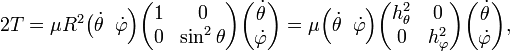$2T = \mu R^2 \big(\dot{\theta}\;\; \dot{\varphi} \Big) \begin{pmatrix} 1 & 0 \\ 0 & \sin^2 \theta \\ \end{pmatrix} \begin{pmatrix} \dot{\theta}\\ \dot{\varphi} \end{pmatrix} = \mu \Big(\dot{\theta}\;\; \dot{\varphi} \Big) \begin{pmatrix} h_\theta^2 & 0 \\ 0 & h_\varphi^2 \\ \end{pmatrix} \begin{pmatrix} \dot{\theta}\\ \dot{\varphi} \end{pmatrix},$

where hθR and hφRsinθ are scale (or Lamé) factors.

Scale factors are of importance for quantum mechanical applications since they enter the Laplacian expressed in curvilinear coordinates. In the case at hand (constant R)$\nabla^2 = \frac{1}{h_\theta h_\varphi}\left[ \frac{\partial}{\partial \theta} \frac{h_\varphi}{h_\theta} \frac{\partial}{\partial \theta} +\frac{\partial}{\partial \varphi} \frac{h_\theta}{h_\varphi} \frac{\partial}{\partial \varphi} \right]= \frac{1}{R^2}\left[\frac{1}{\sin\theta} \frac{\partial}{\partial \theta} \sin\theta \frac{\partial}{\partial \theta} +\frac{1}{\sin^2\theta}\frac{\partial^2}{\partial \varphi^2} \right].$

###  The quantum mechanical linear rigid rotor

The linear rigid rotor model can be used in quantum mechanics to predict the rotational energy of a diatomic molecule. The rotational energy depends on the moment of inertia for the system, I. In the center of mass reference frame, the moment of inertia is equal to:$I = \mu R^2\,$

where μ is the reduced mass of the molecule and R is the distance between the two atoms.

According to quantum mechanics, the energy levels of a system can be determined by solving the Schrödinger equation H Ψ = E Ψ, where Ψ is the wave function and H is the energy (Hamiltonian) operator. For the rigid rotor in a field-free space, the energy operator corresponds to the kinetic energy of the system: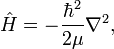$\hat H = - \frac{\hbar^2}{2\mu} \nabla^2,$

where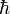$\hbar$ is Planck's constant divided by and$\nabla^2$ is the Laplacian. The Laplacian is given above in terms of spherical polar coordinates. The energy operator written in terms of these coordinates is: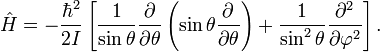$\hat H =- \frac{\hbar^2}{2I} \left [ {1 \over \sin \theta} {\partial \over \partial \theta} \left ( \sin \theta {\partial \over \partial \theta} \right ) + {1 \over {\sin^2 \theta}} {\partial^2 \over \partial \varphi^2} \right].$

This operator appears also in the (θ, φ)-part of the Schrödinger equation of the hydrogen-like atoms,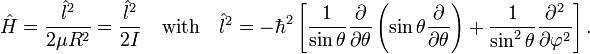$\hat{H} = \frac{\hat{l}^2}{2\mu R^2} = \frac{\hat{l}^2}{2I}\quad\hbox{with}\quad \hat{l}^2 = -\hbar^2 \left [ {1 \over \sin \theta} {\partial \over \partial \theta} \left ( \sin \theta {\partial \over \partial \theta} \right ) + {1 \over {\sin^2 \theta}} {\partial^2 \over \partial \varphi^2} \right].$

The eigenvalue equation becomes$\tfrac{1}{2I}\; \hat{l}^2\;Y_\ell^m(\theta, \varphi ) = \tfrac{1}{2I}\; \hbar^2\ell(\ell+1) \;Y_\ell^m(\theta, \varphi ).$

Here$Y_\ell^m (\theta, \varphi )$ represents a set of functions known as the spherical harmonics. Note that the energy does not depend on the magnetic quantum number$m \,$, in other words, the energy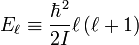$E_\ell \equiv {\hbar^2 \over 2I} \ell \left (\ell+1\right )$

is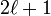$2\ell+1$-fold degenerate: the functions with fixed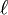$\ell\,$ and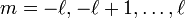$m=-\ell,-\ell+1,\dots,\ell$ have the same energy.

In microwave spectroscopy one prefers using the rotational constant B instead of the inertia moment, and one writes,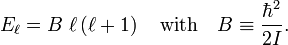$E_\ell = B\; \ell \left (\ell+1\right )\quad \textrm{with}\quad B \equiv \frac{\hbar^2}{2I}.$

In the unit of reciprocal length the rotational constant is,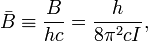$\bar B \equiv \frac{B}{hc} = \frac{h}{8\pi^2cI},$

with c the speed of light. If cgs units are used for h, c, and I,$\bar B$ is expressed in wave numbers, cm−1, a unit that is often used in rotational-vibrational spectroscopy.

Obviously, the rotational constant$\bar B$ depends on the distance R. Often one writes$B_e = \bar B(R_e)$ where Re is the equilibrium value of R (the value for which the interaction energy of the atoms in the rotor has a minimum).

##  Arbitrarily shaped rigid rotor

An arbitrarily shaped rigid rotor is a rigid body of arbitrary shape with its center of mass fixed (or in uniform rectilinear motion) in field-free space${\mathbb{R}^3}$, so that its energy consists only of rotational kinetic energy (plus possibly a constant translational energy that can be ignored). A rigid body can be (partially) characterized by the three eigenvalues of its moment of inertia tensor, which are real nonnegative values known as principal moments of inertia. In microwave spectroscopy—the spectroscopy based on rotational transitions—one usually classifies molecules (seen as rigid rotors) as follows:

• spherical rotors
• symmetric rotors
• oblate symmetric rotors
• prolate symmetric rotors
• asymmetric rotors

This classification depends on the relative magnitudes of the principal moments of inertia.

####  Coordinates of the rigid rotor

Different branches of physics and engineering use different coordinates for the description of the kinematics of a rigid rotor. In molecular physics Euler angles are used almost exclusively. In quantum mechanical applications it is advantageous to use Euler angles in a convention that is a simple extension of spherical polar coordinates.

The first step is the attachment of a right-handed orthonormal frame (3-dimensional system of orthogonal axes) to the rotor (a body-fixed frame) . This frame can be attached arbitrarily to the body, but often one uses the principal axes frame—the normalized eigenvectors of the inertia tensor, which always can be chosen orthonormal, since the tensor is symmetric. When the rotor possesses a symmetry-axis, it usually coincides with one of the principal axes. It is convenient to choose as body-fixed z-axis the highest-order symmetry axis.

One starts by aligning the body-fixed frame with a space-fixed frame (laboratory frame), so that the body-fixed x, y, and z axes coincide with the space-fixed X, Y, and Z axis. Then the body and its frame are rotated actively over a positive angle α around the z-axis (by the right-hand screw rule), which moves the y- to the y'-axis. Thirdly, one rotates the body and its frame over a positive angle β around the y'-axis. The z-axis of the body-fixed frame has after these two rotations the longitudinal angle α (commonly designated by φ) and the colatitude angle β (commonly designated by θ), both with respect to the space-fixed frame. If the rotor were cylindrical symmetric around its body-fixed z-axis, like the linear rigid rotor, its orientation in space would be unambiguously specified at this point.

If the body lacks cylinder (axial) symmetry, a last rotation around its z-axis (which has polar coordinates β and α) is necessary to specify its orientation completely. Traditionally the last rotation angle is called γ.

The convention for Euler angles used here is known as the z' − y' − z convention; it can be shown that it is equivalent to the zyz convention in which the order of rotations is reversed.

The total matrix of the three consecutive rotations is the product$\mathbf{R}(\alpha,\beta,\gamma)= \begin{pmatrix} \cos\alpha & -\sin\alpha & 0 \\ \sin\alpha & \cos\alpha & 0 \\ 0 & 0 & 1 \end{pmatrix} \begin{pmatrix} \cos\beta & 0 & \sin\beta \\ 0 & 1 & 0 \\ -\sin\beta & 0 & \cos\beta \\ \end{pmatrix} \begin{pmatrix} \cos\gamma & -\sin\gamma & 0 \\ \sin\gamma & \cos\gamma & 0 \\ 0 & 0 & 1 \end{pmatrix}$

Let r(0) be the coordinate vector with respect to the body-fixed frame of an arbitrary point ℘ of the body. We call the three elements of r(0) the "body-fixed coordinates" of ℘. Initially r(0) is also the space-fixed coordinate vector of ℘. Upon rotation of the body (including its frame and its point ℘), the body-fixed coordinates of ℘ do not change, but the space-fixed coordinate vector of ℘ becomes,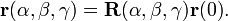$\mathbf{r}(\alpha,\beta,\gamma)= \mathbf{R}(\alpha,\beta,\gamma)\mathbf{r}(0).$

In particular, if ℘ is initially on the space-fixed Z-axis, it has the space-fixed coordinates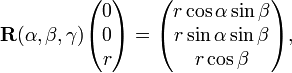$\mathbf{R}(\alpha,\beta,\gamma) \begin{pmatrix} 0 \\ 0 \\ r \\ \end{pmatrix}= \begin{pmatrix} r \cos\alpha\sin\beta \\ r \sin\alpha \sin\beta \\ r \cos\beta \\ \end{pmatrix},$

which shows the correspondence with the spherical polar coordinates.

Knowledge of the Euler angles as function of time t and the initial coordinate vector r(0) determine the kinematics of the rigid rotor.

####  Classical kinetic energy

The following is a generalization of the well-known special case of the rotational energy of an object that rotates around one axis.

It will be assumed from here on that the body-fixed frame is a principal axes frame; it diagonalizes the instantaneous inertia tensor$\mathbf{I}(t)$ (expressed with respect to the space-fixed frame), i.e.,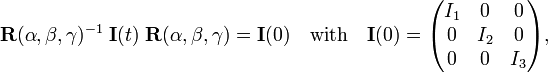$\mathbf{R}(\alpha,\beta,\gamma)^{-1}\; \mathbf{I}(t)\; \mathbf{R}(\alpha,\beta,\gamma) = \mathbf{I}(0)\quad\hbox{with}\quad \mathbf{I}(0) = \begin{pmatrix} I_1 & 0 & 0 \\ 0 & I_2 & 0 \\ 0 & 0 & I_3 \\ \end{pmatrix},$

where the Euler angles are time-dependent and in fact determine the time dependence of$\mathbf{I}(t)$ by the inverse of this equation. This notation implies that at t = 0 the Euler angles are zero, so that at t = 0 the body-fixed frame coincides with the space-fixed frame.

The classical kinetic energy T of the rigid rotor can be expressed in different ways:

• as a function of angular velocity
• in Lagrangian form
• as a function of angular momentum
• in Hamiltonian form.

Since each of these forms has its use and can be found in textbooks we will present all of them.

#####  Angular velocity form

As a function of angular velocity T reads,$T = \frac{1}{2} \left[ I_1 \omega_x^2 + I_2 \omega_y^2+ I_3 \omega_z^2 \right]$

with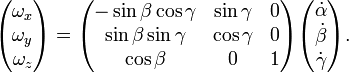$\begin{pmatrix} \omega_x \\ \omega_y \\ \omega_z \\ \end{pmatrix} = \begin{pmatrix} -\sin\beta\cos\gamma & \sin\gamma & 0 \\ \sin\beta\sin\gamma & \cos\gamma & 0 \\ \cos\beta & 0 & 1 \\ \end{pmatrix} \begin{pmatrix} \dot{\alpha} \\ \dot{\beta} \\ \dot{\gamma} \\ \end{pmatrix}.$

The vector ω = (ωx, ωy, ωz) contains the components of the angular velocity of the rotor expressed with respect to the body-fixed frame. It can be shown that ω is not the time derivative of any vector, in contrast to the usual definition of velocity. The dots over the time-dependent Euler angles indicate time derivatives.

#####  Lagrange form

Backsubstitution of the expression of ω into T gives the kinetic energy in Lagrange form (as a function of the time derivatives of the Euler angles). In matrix-vector notation,$2 T = \begin{pmatrix} \dot{\alpha} & \dot{\beta} & \dot{\gamma} \end{pmatrix} \; \mathbf{g} \; \begin{pmatrix} \dot{\alpha} \\ \dot{\beta} \\ \dot{\gamma}\\ \end{pmatrix},$

where$\mathbf{g}$ is the metric tensor associated with Euler angles—a non-orthogonal system of curvilinear coordinates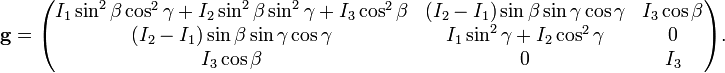$\mathbf{g}= \begin{pmatrix} I_1 \sin^2\beta \cos^2\gamma+I_2\sin^2\beta\sin^2\gamma+I_3\cos^2\beta & (I_2-I_1) \sin\beta\sin\gamma\cos\gamma & I_3\cos\beta \\ (I_2-I_1) \sin\beta\sin\gamma\cos\gamma & I_1\sin^2\gamma+I_2\cos^2\gamma & 0 \\ I_3\cos\beta & 0 & I_3 \\ \end{pmatrix}.$
#####  Angular momentum form

Often the kinetic energy is written as a function of the angular momentum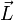$\vec{L}$ of the rigid rotor. This vector is a conserved (time-independent) quantity. With respect to the body-fixed frame it has the components$\mathbf{L}$, which can be shown to be related to the angular velocity,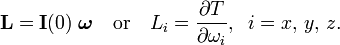$\mathbf{L} = \mathbf{I}(0)\; \boldsymbol{\omega}\quad\hbox{or}\quad L_i = \frac{\partial T}{\partial\omega_i},\;\; i=x,\,y,\,z.$

Since the body-fixed frame moves (depends on time) these components are not time independent. If we were to represent$\vec{L}$ with respect to the stationary space-fixed frame, we would find time independent expressions for its components. The kinetic energy is given by$T = \frac{1}{2} \left[ \frac{L_x^2}{I_1} + \frac{L_y^2}{I_2}+ \frac{L_z^2}{I_3}\right].$
#####  Hamilton form

The Hamilton form of the kinetic energy is written in terms of generalized momenta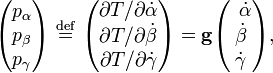$\begin{pmatrix} p_\alpha \\ p_\beta \\ p_\gamma \\ \end{pmatrix} \ \stackrel{\mathrm{def}}{=}\ \begin{pmatrix} \partial T/{\partial \dot{\alpha}}\\ \partial T/{\partial \dot{\beta}} \\ \partial T/{\partial \dot{\gamma}} \\ \end{pmatrix} = \mathbf{g} \begin{pmatrix} \; \, \dot{\alpha} \\ \dot{\beta} \\ \dot{\gamma}\\ \end{pmatrix},$

where it is used that the$\mathbf{g}$ is symmetric. In Hamilton form the kinetic energy is,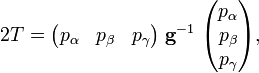$2 T = \begin{pmatrix} p_{\alpha} & p_{\beta} & p_{\gamma} \end{pmatrix} \; \mathbf{g}^{-1} \; \begin{pmatrix} p_{\alpha} \\ p_{\beta} \\ p_{\gamma}\\ \end{pmatrix},$

with the inverse metric tensor given by${\sin^2\beta}\;\;\mathbf{g}^{-1}=$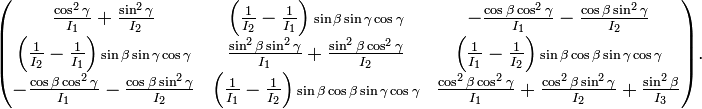$\begin{pmatrix} \frac{\cos^2\gamma}{I_1}+\frac{\sin^2\gamma}{I_2} & \left(\frac{1}{I_2}-\frac{1}{I_1}\right){\sin\beta\sin\gamma\cos\gamma}& -\frac{\cos\beta\cos^2\gamma}{I_1}-\frac{\cos\beta\sin^2\gamma}{I_2} \\ \left(\frac{1}{I_2}-\frac{1}{I_1}\right){\sin\beta\sin\gamma\cos\gamma}& \frac{\sin^2\beta\sin^2\gamma}{I_1}+\frac{\sin^2\beta\cos^2\gamma}{I_2} & \left(\frac{1}{I_1}-\frac{1}{I_2}\right){\sin\beta\cos\beta\sin\gamma\cos\gamma}\\ -\frac{\cos\beta\cos^2\gamma}{I_1}-\frac{\cos\beta\sin^2\gamma}{I_2} & \left(\frac{1}{I_1}-\frac{1}{I_2}\right){\sin\beta\cos\beta\sin\gamma\cos\gamma} & \frac{\cos^2\beta\cos^2\gamma}{I_1}+ \frac{\cos^2\beta\sin^2\gamma}{I_2}+\frac{\sin^2\beta}{I_3} \\ \end{pmatrix}.$

This inverse tensor is needed to obtain the Laplace-Beltrami operator, which (multiplied by$-\hbar^2$) gives the quantum mechanical energy operator of the rigid rotor.

The classical Hamiltonian given above can be rewritten to the following expression, which is needed in the phase integral arising in the classical statistical mechanics of rigid rotors,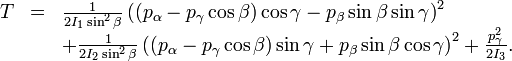$\begin{array}{lcl} T &=& \frac{1}{2I_1 \sin^2\beta} \left( (p_\alpha- p_\gamma\cos\beta)\cos\gamma -p_\beta \sin\beta\sin\gamma \right)^2 \\ &&+ \frac{1}{2I_2 \sin^2\beta} \left( (p_\alpha- p_\gamma\cos\beta)\sin\gamma +p_\beta \sin\beta\cos\gamma \right)^2 + \frac{p_\gamma^2}{2I_3}. \\ \end{array}$

###  Quantum mechanical rigid rotor

As usual quantization is performed by the replacement of the generalized momenta by operators that give first derivatives with respect to its canonically conjugate variables (positions). Thus,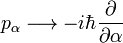$p_\alpha \longrightarrow -i \hbar \frac{\partial}{\partial \alpha}$

and similarly for pβ and pγ. It is remarkable that this rule replaces the fairly complicated function pα of all three Euler angles, time derivatives of Euler angles, and inertia moments (characterizing the rigid rotor) by a simple differential operator that does not depend on time or inertia moments and differentiates to one Euler angle only.

The quantization rule is sufficient to obtain the operators that correspond with the classical angular momenta. There are two kinds: space-fixed and body-fixed angular momentum operators. Both are vector operators, i.e., both have three components that transform as vector components among themselves upon rotation of the space-fixed and the body-fixed frame, respectively. The explicit form of the rigid rotor angular momentum operators is given here (but beware, they must be multiplied with$\hbar$). The body-fixed angular momentum operators are written as$\hat{\mathcal{P}}_i$. They satisfy anomalous commutation relations.

The quantization rule is not sufficient to obtain the kinetic energy operator from the classical Hamiltonian. Since classically pβ commutes with cosβ and sinβ and the reciprocals of these functions, the position of these trigonometric functions in the classical Hamiltonian is arbitrary. After quantization the commutation no longer holds and the order of operators and functions in the Hamiltonian (energy operator) becomes a point of concern. Podolsky proposed in 1928 that the Laplace-Beltrami operator (times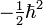$-\tfrac{1}{2}\hbar^2$) has the appropriate form for the quantum mechanical kinetic energy operator. This operator has the general form (summation convention: sum over repeated indices—in this case over the three Euler angles q1 ≡ α, q2 ≡ β, q3 ≡ γ),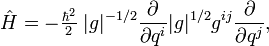$\hat{H} = - \tfrac{\hbar^2}{2}\;|g|^{-1/2} \frac{\partial}{\partial q^i} |g|^{1/2} g^{ij} \frac{\partial}{\partial q^j},$

where | g | is the determinant of the g-tensor:$|g| = I_1\, I_2\, I_3\, \sin^2 \beta \quad \hbox{and}\quad g^{ij} = (\mathbf{g}^{-1})_{ij}.$

Given the inverse of the metric tensor above, the explicit form of the kinetic energy operator in terms of Euler angles follows by simple substitution.

It can be shown that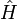$\hat{H}$ can be expressed in body-fixed angular momentum operators (in this proof one must carefully commute differential operators with trigonometric functions). The result has the same appearance as the classical formula expressed in body-fixed coordinates,$\hat{H} = \tfrac{1}{2}\left[ \frac{\mathcal{P}_x^2}{I_1}+ \frac{\mathcal{P}_y^2}{I_2}+ \frac{\mathcal{P}_z^2}{I_3} \right].$

The action of the$\hat{\mathcal{P}}_i$ on the Wigner D-matrix is simple. In particular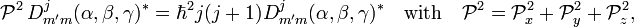$\mathcal{P}^2\, D^j_{m'm}(\alpha,\beta,\gamma)^* = \hbar^2 j(j+1) D^j_{m'm}(\alpha,\beta,\gamma)^* \quad\hbox{with}\quad \mathcal{P}^2= \mathcal{P}^2_x + \mathcal{P}_y^2+ \mathcal{P}_z^2,$

so that the Schrödinger equation for the spherical rotor (I1 = I2 = I3) is solved with the (2j+1)2 degenerate energy equal to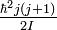$\tfrac{\hbar^2 j(j+1)}{2I}$ and Wigner D-matrix elements as eigenfunctions.

#### Schrödinger equation for symmetric top

The symmetric top (= symmetric rotor) is characterized by two equal principal moments of inertia. It is a prolate (cigar shaped) top if I3 < I1 = I2. In this case we write the Hamiltonian as$\hat{H} = \tfrac{1}{2}\left[ \frac{\mathcal{P}^2}{I_1}+ \mathcal{P}_z^2\Big(\frac{1}{I_3} -\frac{1}{I_1} \Big) \right].$

Introducing the angular momentum operators in their differential operator form, we obtain the Schrödinger equation as it was solved for the first time by Kronig and Rabi. The symmetric top is one of the few cases where the Schrödinger equation can be solved analytically. All these cases were solved within a year of the formulation of the Schrödinger equation.

Knowing the form of the Hamiltonian in terms of angular momentum operators, and knowing the relation for Wigner D-matrices,$\mathcal{P}_z^2\, D^j_{m k}(\alpha,\beta,\gamma)^* = \hbar^2 k^2\, D^j_{m k}(\alpha,\beta,\gamma)^*,$

the Schrödinger equation does not any longer have to be solved from scratch, as Kronig and Rabi had to do.

Indeed,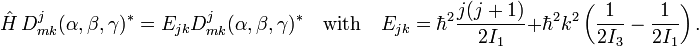$\hat{H}\,D^j_{m k}(\alpha,\beta,\gamma)^* = E_{jk} D^j_{m k}(\alpha,\beta,\gamma)^* \quad \hbox{with}\quad E_{jk} = \hbar^2\frac{j(j+1)}{2I_1} + \hbar^2 k^2\left(\frac{1}{2I_3}-\frac{1}{2I_1}\right).$

The eigenvalue Ej0 is (2j + 1)-fold degenerate, because all eigenfunctions with m = − j, −j + 1, ..., j have the same eigenvalue. Since k appears squared in the energy expression, the energies with k ≠ 0 are 2(2j + 1)-fold degenerate. The complex conjugates of the Wigner D-matrix elements are the corresponding eigenfunctions, that is, they are the wave functions of the symmetric top.

The asymmetric top problem ($I_1 \ne I_2 \ne I_3$) is not exactly soluble.